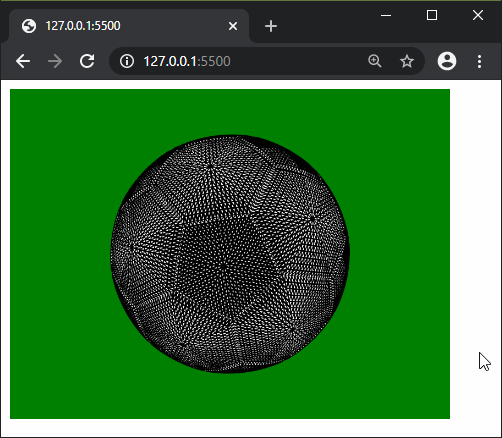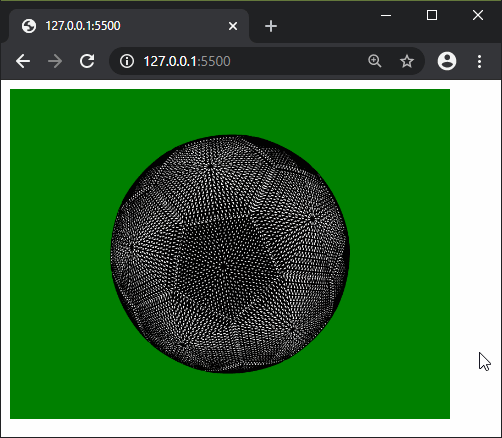# p5.js | loadModel() Function

• Last Updated : 20 Jun, 2022

The loadModel() function is used to load a 3d model from file, and return it as a p5.Geometry Object. This model can be loaded with the formats .obj or .stl. The OBJ and STL loaded files do not have any sense of scale, hence it may be required to use the normalized parameter so that the model is automatically scaled to the correct size. The scale() function can be used once the model is loaded to make further changes to the size of the model. This method is asynchronous in nature and therefore it may not finish before the model can be used. It is hence advised to load the model in the preload() function.

Syntax:

`loadModel( path, normalize, [successCallback], [failureCallback] )`

or

`loadModel(path, [successCallback], [failureCallback])`

Parameters: This function accept four parameters as mentioned above and described below:

• path: It is a string which denotes the path from where the model has to be loaded.
• normalize: It is a boolean value which determines if the loaded model would be scaled to standardized size when loading. It is an optional parameter.
• successCallback: This is a function which is called if the model is loaded successfully. The loaded model is passed as a parameter. It is an optional parameter.
• failureCallback: This is a function which is called if the model does not load due to any error. The error event is passed as a parameter. It is an optional parameter.

Return Value: It returns a p5.Geometry object with the given model.

Below programs illustrate the loadModel() function in p5.js:

Example 1: This example shows how the model is loaded in the preload() function.

## javascript

 `let newObj;`   `function` `preload() {` `  ``newObj = loadModel(``'models/ball.obj'``, ``true``);` `}`   `function` `setup() {` `  ``createCanvas(400, 300, WEBGL);` `}`   `function` `draw() {` `  ``background(``'green'``);`   `  ``rotateZ(frameCount * 0.01);` `  ``rotateX(frameCount * 0.01);`   `  ``model(newObj);` `}`

Output:Example 2: This example shows how the callbacks can be used to load and use the model.

## javascript

 `let newModel;`   `function` `setup() {` `  ``createCanvas(400, 300, WEBGL);` `  ``noLoop();` `  ``loadModel(``'models/ball.obj'``, ``true``, modelLoaded, loadFailed);` `}`   `function` `draw() {` `  ``background(``'green'``);`   `  ``rotateZ(frameCount * 0.01);` `  ``rotateX(frameCount * 0.01);` `  ``model(newModel);`   `  ``loop();` `}`   `function` `modelLoaded(modelObj) {` `  ``newModel = modelObj;` `  ``loop();` `}`   `function` `loadFailed(error) {` `  ``print("The model failed to load", error);` `}`

Output:My Personal Notes arrow_drop_up
Recommended Articles
Page :